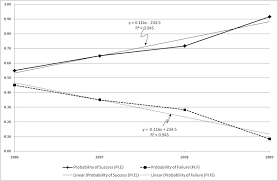## How to Calculate and Solve for Success and Failure | ProbabilityThe image above represents success and failure.

To compute for success and failure, two essential parameters are needed and these parameters are and N.

The formula for calculating success:

P(success) = x N

Where;

x = Number of successes
N = Total Number of Trials or Items

Let’s solve an example;
Find the success when the x is 12 and N is 14?

This implies that;

x = Number of successes = 12
N = Total Number of Trials or Items = 14

P(success) = x N
P(success) = 12 14
Dividing the numerator and denominator by 2
P(success) = 6 7
P(success) = 0.857

Therefore, the success is 0.857.